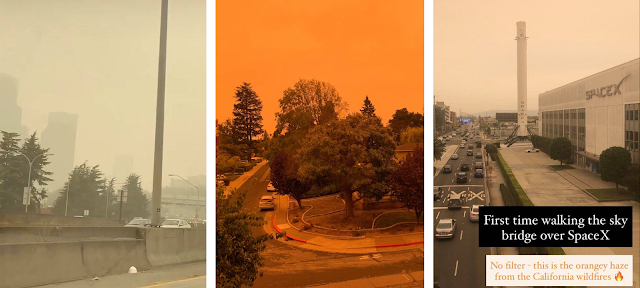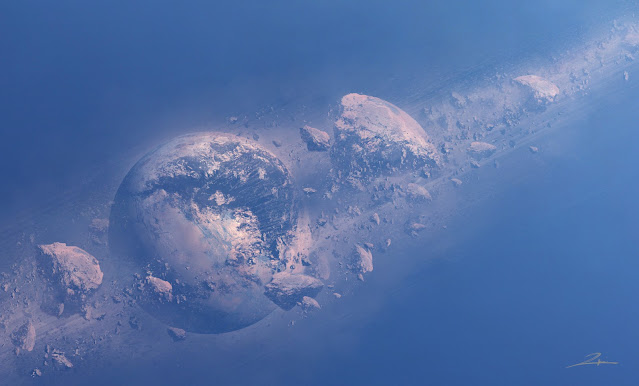### Igniting the Atmosphere with Moon Rocks

"The Moon blew up without warning and for no apparent reason. It was waxing, only one day short of full. The time was 05:03:12 UTC. Later it would be designated A+0.0.0, or simply Zero" - Seveneves, Neal Stephenson
_______________________________________________________________

As if 2020 wasn't going disastrously enough already with COVID-19, the enormous wildfires engulfing the West Coast have left multiple cities under an apocalyptic haze of dust and smoke. It's as if the sky were on fire, or if Earth and Mars inexplicably switched atmospheres. The unsightly view out my window got me thinking about the latest book I've been reading to pass the time under quarantine, since we all know I can't resist a good space-themed hard sci-fi adventure! Neal Stephenson's novel Seveneves imagines a near future where the Moon inexplicably shatters into enormous fragments, unleashing an onslaught of lunar meteors that will soon incinerate the atmosphere and decimate the Earth. In the intervening time, the nations of the world must throw everything they've got into beefing up the International Space Station and launching as many people into orbit as possible to preserve the human race, before the world is engulfed in fire and furyThe orange sky over Seattle, San Francisco, and Los Angeles (pics by my sister, my friend, and myself)

Similar to The Martian, which received acclaim not just for its compelling story and witty humor, but also for its precise attention to scientific accuracy, Seveneves does an excellent job of weaving astrophysics and orbital mechanics into an exciting plot that assumes the reader already knows a thing or two about space. And in instances that run counter to people's intuition, the story provides fascinating surprises both for the reader and the characters. For example, when the Moon initially explodes, it breaks apart into seven pieces that remain clustered around the former Moon's center of gravity. Despite the initial shock, everyone on Earth assumes the new fragments are stable and even gives them cute nicknames: Potatohead, Mr. Spinny, Acorn, Peach Pit, Scoop, Big Boy, and Kidney Bean. But a week later when two of the fragments collide and shatter into innumerable pieces, the world realizes the apocalypse is nigh. In such a chaotic gravitational system, collisions will start slow but grow exponentially as more and more fragments are created, until eventually an enormous firestorm of bolides crashes down on Earth. With the help of mathematical models, the time frame is set: two years until the "White Sky" and the "Hard Rain" - the race is on to save humanity!If the moon exploded tomorrow, I wouldn't even be surprised anymore. 2020 has been that kind of year...

I won't spoil the book for you, it's really a fantastic read. But I'll give you a little excerpt of when the "Hard Rain" comes at the end of the two years and ravages the Earth, since it got me thinking about a cool math problem I'd like to try calculate:

"Dinah wiped her eyes and looked out her window to see Earth encircled by a belt of fire. The trails of the incoming meteorites, once a pattern of bright scratches in the air, had merged into a blinding continuum of superheated air that had set fire to anything on the surface capable of burning. Since more of the rocks were coming in around the equator, the belt of radiance and fire was brightest there; but north and south of it, long swaths of the surface were aflame"Better hope to God we never see this sight in the skies above Earth | Credit: u/Dodgiestyle on Reddit

So here's the question I asked myself - knowing that the average temperature of Earth's atmosphere is about $14^{\circ}C$  ($57^{\circ}F$), could I calculate a SUPER ROUGH estimate of what the Earth's atmospheric temperature would be after bombardment by the Lunar Hard Rain? (and by super rough, I really mean super rough, ballpark order of magnitude). This calculation will draw on my high school knowledge of thermodynamics - hopefully terms like specific heat capacity and heat of vaporization ring a bell!

#### And I thought my hometown of San Antonio was hot already...

We first need to estimate the enormous heat released by the kinetic energy of falling moon rocks being converted to thermal energy by friction against the atmosphere. Knowing that the mass of the entire Moon is $7.3 \cdot 10^{22}kg$, if we assume a mere 5% of lunar material ultimately falls to Earth (the book states that the majority of the moon stays in orbit and will eventually form a ring around the Earth, far into the future), that gives us $3.7 \cdot 10^{21}kg$ of meteors

What would be the starting temperature of the moon meteors before they fall to Earth? Because the Moon has no atmosphere, the Apollo astronauts faced large temperature variations between +/- $120^{\circ}C$ in sunlight and shadow. This seems like a big range, but when you consider that during atmospheric entry, these moon rocks will reach temperatures approaching $3,000^{\circ}C$, whether we pick the upper or lower end of the range is relatively negligible, so we'll split the difference and call it $0^{\circ}C$

So how much thermal energy will be released as $3.7 \cdot 10^{21}kg$ of moon rock is heated from $0^{\circ}C$ to $3,000^{\circ}C$, knowing that the solid rocks will vaporize in the process? There are two concepts you need to recall from high school thermodynamics:

• Every substance has a physical property known as specific heat capacity, which states how much thermal energy it takes to raise the temperature of 1 gram of that material by 1 degree Celsius
• Every substance has a heat of fusion and a heat of vaporization, which states how much thermal energy it takes to convert it from solid to liquid and liquid to gas at its respective melting and boiling points. For example, a kilogram of ice and a kilogram of liquid water might both be at $0^{\circ}C$, but the kilogram of water has more thermal energy because it absorbed the heat of fusion needed to melt that ice into waterThermodynamics was one of my best units in high school physics! It was optics and light ray diagrams that wrecked me :(

It's easy to find these values for common substances like water, but not so much for trying to vaporize rocks (like seriously, why on Earth would anyone be trying to do that?). But here are my best estimates for the values I'll be using:

• The two most common compounds that make up the moon are silicon dioxide and aluminum oxide. To keep our calculation simple, I'm going to split the differences in the two compounds' chemical properties and use a melting point of $1,800^{\circ}C$ and a boiling point of $2,950^{\circ}C$
• I found estimates for their specific heat capacities here and here. Once again, I'm going to split the difference between the two and call it at $700 \thinspace J/kg \cdot C$. I'm also going to make the assumption that the specific heat capacity will be the same in solid, liquid, and gaseous states (not a great assumption, since I know ice, water, and steam have rather different specific heat capacities, but there's no way I'm going to find the specific heat capacity of liquid silicon dioxide)
• Heat of fusion and vaporization was an exceptionally challenging estimate to find, but my best sources are here and here. Minerals on the Earth and on the Moon aren't that different, so I'll say $600 \thinspace J/g$ and $4,500 \thinspace J/g$ for fusion and vaporization, respectively

Great, now we have all our inputs. Here are the two formulas we'll be using

• Temperature change in the same state: $Q = mC\Delta T$
Thermal energy = mass * specific heat * change in temperature
• Change of state (melting/boiling): $Q = mH_f$   or   $Q = mH_v$
Thermal energy = mass * heat of fusion/vaporization

Step 1 (solid): $Q = (3.7 \cdot 10^{21}) \cdot 700 \cdot 1800 = 4.7 \cdot 10^{27} \thinspace J$
Step 2 (melting): $Q = (3.7 \cdot 10^{21}) \cdot (600 \cdot 1000) = 2.2 \cdot 10^{27} \thinspace J$
Step 3 (liquid): $Q = (3.7 \cdot 10^{21}) \cdot 700 \cdot (2950-1800) = 3.0 \cdot 10^{27} \thinspace J$
Step 4 (vaporizing): $Q = (3.7 \cdot 10^{21}) \cdot (4500 \cdot 1000) = 1.7 \cdot 10^{28} \thinspace J$
Step 5 (gas): $Q = (3.7 \cdot 10^{21}) \cdot 700 \cdot (3000-2950) = 1.3 \cdot 10^{26} \thinspace J$

Add it all up, and we get $2.7 \cdot 10^{28} \thinspace J$ of thermal energy released as all our moon rocks burn up in the Earth

Now we need to apply all the heat on Earth's current atmosphere. The mass of the entirety of Earth's atmosphere is $5.2 \cdot 10^{18}kg$, and the specific heat capacity of air is about $1000 \thinspace J/kg \cdot C$

Taking our formula $Q = mC\Delta T$, we get $2.7 \cdot 10^{28} = 5.2 \cdot 10^{18} \cdot 1000 \cdot \Delta T$

Solve for $\Delta T$, and the answer is problematic. I get a temperature increase of over 5 million degrees Celsius! This is obviously nonsensical, the problem is we assumed the entirety of the thermal energy released by the lunar meteors is transferred only to the Earth's atmospheric gas, and not to the Earth itself (ie the solid Earth will heat up substantially too). But hey, it was a fun calculation nonetheless, and I hope it demonstrates how doomed we would be if the Moon blew up